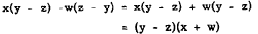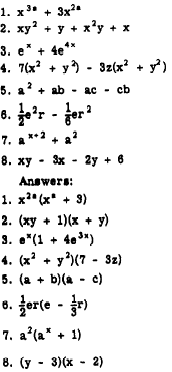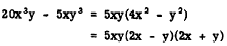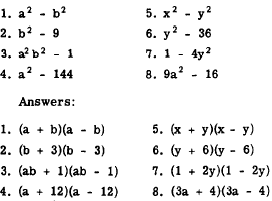Binomial FormsCustom SearchBINOMIAL FORM The distinctions between monomial, binomial, and trinomial factors are discussed in detail in chapter 9 of this course. An expression such as a(x + y) + b(x + y) has a common factor in binomial form. The factor (x + y) can be removed from both terms, with the following result: a(x + y) + b(x + y) - (x + y)(a + b) Sometime0 it I6 easier to see this if a single letter is substituted temporarily for the binomial, Thus, let (x + y) - n, so that a(x + y) + b(x + y) reducer to (an + bn). The factored form is n(a + b), which becomes (x + y)(a + b) when n is replaced by its equal, (x + y). Another form of this type is x(y - 2) - w(a - y). Notice that this expression could be factored easily if the binomial in the Second term were (y-z).  We can show that -w(z - y) is equalivalent to w(z-y), as follows:Substituting +w(y - z) for -w(z - y) in the original expression, we may now factor as follows:In factoring an expression such as ax + bx + ay + by, common monomial factors are removed first, as follows:         ax + bx + ay + by = x(a + b) + y(a + b) Having removed the common monomial factors, we then remove the common binomial factor to obtain (a + b)(x + y). Notice that we could have rewritten the expression as ax + ay + bx + by, based on the commutative law of addition, which states that the sum of two or more terms is the same regardless of the order in which they are arranged. The first step in factoring would then produce a(x + y) + b(x + y) and the final form would be (x + y)(a + b). This is equivalent to (a + b)(x + y), by the commutative law of multiplication, which states that the product of two or more factors is the same regardless of the order in which they are arranged. Practice problems. Factor each of the following:BINOMIAL FACTORS After any common factor has been removed from a polynomial, the remaining polynomial factor must be examined further for other factors. Skill in factoring is principally the ability to recognize certain types of products such as the square of a sum or difference. Therefore, it is important to be familiar with the special products discussed in chapter 9. DIFFERENCE OF TWO SQUARES In chapter 9 we learned that the product of the sum and difference of two numbers is the difference of their squares. Thus, (a + b)(a - b) = a2 - b2. Conversely, if a binomial is the difference of two squares, its factors are the sum and difference of the square roots. For example, in 9a2 - 4b2 both 9a2 and 4b2 are perfect squares. The square roots are 3a and 2b, respectively. Connect these square roots with a plus sign to get one factor of 9a2 - 4b2 and with a minus sign to get the other factor. The two binomial factors are 3a - 2b and 3a + 2b. Therefore, factored completely, the binomial can be written as follows:         9a2 - 4b2 = (3a - 2b)(3a + 2b) We may check to see if these factors are correct by multiplying them together to see if their product is the original binomial. The expression 20x y3 - 5xy3 reduces to the difference of two squares after the common factor 5xy is removed. Completely factored, this expression produces the following:Other examples that show the difference of two squares in factored form are as follows:Practice problems: Factor each of the following:Integrated Publishing, Inc. - A (SDVOSB) Service Disabled Veteran Owned Small Business